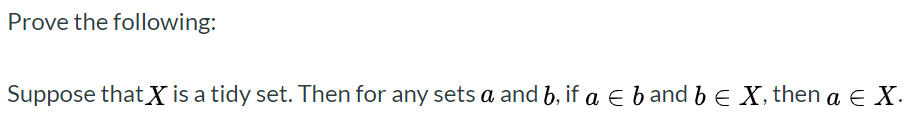# Prove the following:Suppose that X is a tidy set. Then for any sets a and b, if a e b and b E X, then a E X

Question
21 views

Prove the following:

Suppose thatX is a tidy set. Then for any sets a and b, if a ∈ b and b ∈ X, then a ∈ X.help_outlineImage TranscriptioncloseProve the following: Suppose that X is a tidy set. Then for any sets a and b, if a e b and b E X, then a E X fullscreen
check_circle

Step 1

Consider,

X be a tidy set that represent all data set that each column is a variable and each raw is known as observation.

Step 2

Suppose,

X = {1, 2, 3, 4, 5, 6, 7, 8}

b = {1 ,2, ...

### Want to see the full answer?

See Solution

#### Want to see this answer and more?

Solutions are written by subject experts who are available 24/7. Questions are typically answered within 1 hour.*

See Solution
*Response times may vary by subject and question.
Tagged in

### Other